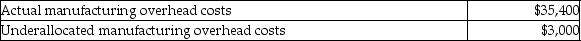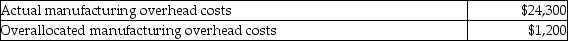# [Solved] Calculate the Unknowns for the Following Independent Situations

Question 140
Essay

## Calculate the unknowns for the following independent situations.1.Selected data for Lion Corporation:Allocated manufacturing overhead is based on 50% of direct labor cost.a.Calculate the allocated manufacturing overhead cost.b.Calculate the direct labor cost.2.Selected data for Tiger Corporation:Manufacturing overhead is allocated at \$15 per machine hour.a.Calculate the allocated manufacturing overhead cost.b.Calculate the number of machine hours incurred.

10+ million students use Quizplus to study and prepare for their homework, quizzes and exams through 20m+ questions in 300k quizzes.

Explore our library and get Accounting Homework Help with various study sets and a huge amount of quizzes and questions

3.5K

Study sets

66.5K

Quizzes

5.9M

Questions

Upload material to get free accessInvite a friend and get free accessSubscribe and get an instant access